Dr. Herrick's CH223 Pressure Quiz 1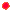Chemical Equilibrium Copyright D. Herrick20 Questions from past exams. Practice for speed.  Aim for 2 minutes per problem.
 Ideal gas constant:  R = 0.08206 L-atm/mol-K = 8.314 J/mol-K
 1 Determine the standard Kc expression for the reaction    C(s) + 2 N2O(g)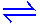CO2(g) + 2 N2(g)
 A)   [C][N2O]2 F)   [CO2]1/2[N2] / [N2O] B)   [N2]2[CO2] / [N2O]2 G)  [N2]2[CO2] / [N2O]2[C] C)   [CO2][N2]2 H)  2[N2] - [CO2] - 2[N2O] D)  1/[C] I)    [N2O]-1/2[CO2][N2]1/2 E)   [N2O]2 [CO2][N2]2 J)   [C]-1[N2]2[CO2][N2O]-2
 2 An equilibrium mixture for the reaction 2A(g) + 3B(g)C(g) + 5D(g) has 0.250 M A, 0.125 M B, 2.000 M C and 0.500 M D. The value of Kc is:
 A) 128 B) 0.063 C) 512 D) 32.000 E) 5.143 F) 256 G) 266.7 H) 1.000 I) 2.000 J)  zero
 3 3 mol of A and 2 mol of B placed in a 1-liter vessel react to equilibrium according to the gas-phase reaction     2 A + B3 C If "x" denotes the mols of B reacted, then the expression for Kc is:
 A)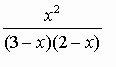D)B)E)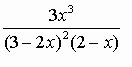C)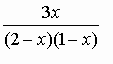F)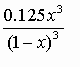4 The reaction 2A(g)B(g) has KP = 16.  If the equilibrium pressure of B is 9 atm, the pressure of A (atm) is:
 A) 1296 B) 0.56 C) 144 D) 1.33 E)  0.50 F)  6.00 G)  0.38 H) 8.25 I) 1.50 J)  0.75
 5 Determine the concentration (M) of NO2 after 1.00 mol of N2O4 placed in a 1.00-liter flask comes to equilibrium according to the reaction    N2O4(g)2 NO2(g) ,   Kc = 2.000
 A) 0.618 B) 1.125 C) 2.000 D) 0.732 E)  1.000 F) 0.000 G) 0.500 H) 1.236 I) 1.414 J) 0.250
 6 Use the reactions  H2S + H2OH3O+ + HS- ,   K1 = 1 × 10-7    HS- + H2OH3O+ + S2-  ,   K2 = 1 × 10-17  to find K for  H2S + 2H2O2H3O+ + S2-
 A) 1 × 1010 D) 1 × 10-3 B) 1 × 1024 E) 1 × 10-10 C) 1 × 103 F) 1 × 10-247 Use the reactions  HCN + H2OH3O+ + CN- ,   K1 = 1 × 10-10    2H2OH3O+ + OH- ,   K2 = 1 × 10-14  to find K for the acid-base neutralization  OH- + HCNH2O + CN-
 A) 1 × 1014 D) 1 × 10-4 B) 1 × 1024 E) 1 × 10-14 C) 1 × 104 F) 1 × 10-24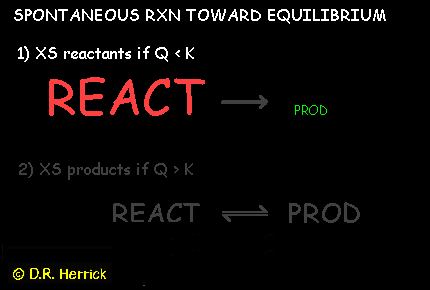8 The gas phase equilibrium reaction 3 H2(g)  +  N2(g)2 NH3(g)  has Kc = 0.0024 at 1000K.   When 2 mol of H2, 5 mol of N2, and 3 mol of NH3 are mixed in a 100-liter vessel at 1000K the result is:
 A) produce more hydrogen and nitrogen from ammonia. B) convert hydrogen and nitrogen into more ammonia. C) no reaction, the starting mixture is at equilibrium. D) the concentration of each reactant and product doubles. E) the concentration of each reactant and product is halved.
 9 Given the equilibrium constants for the reactions   4Cu(s) + O2(g)2Cu2O(s),  K1   2CuO(s)Cu2O(s) + 0.5 O2(g),  K2 what is K for the reaction  2Cu(s) + O2(g)2CuO(s) ?
 A) K11/2 × K2 B) K1 × K21/2 C) K21/2 / K1 D) K11/2 / K2 E) K1 × K2Questions 10-14 consider an equilibrium mixture for  the heterogeneous reaction  2 C(s)  +  O2(g)2 CO(g)     (exothermic)
 A) 2 C +  O2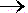2 CO B) 2 C +  O2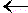2 CO C) no shift
 11 How does the equilibrium shift when the total pressure is increased?
 A) 2 C +  O22 CO B) 2 C +  O22 CO C) no shift
 12 How does the equilibrium shift when the temperature is increased?
 A) 2 C +  O22 CO B) 2 C +  O22 CO C) no shift
 A) 2 C +  O22 CO B) 2 C +  O22 CO C) no shift
 14 The conversion  Kp = Kc (RT)b  for this reaction has b=
 A) 5 B) 4 C) 3 D) 2 E)  1 F) 0 G) -1 H) -2 I) -3 J) -4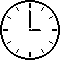15 Which change would cause the following equilibrium to shift to the right?  2NOBr(g)2NO(g) + Br2(g),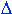Ho  = 30 kJ
 A) Decrease the volume of the reaction vessel. B) Add some NO. C) Remove some NOBr. D) Add Br2. E) Increase the temperature.
 16 Determine Kc for the heterogeneous equilibrium at 500K:  A(s) + 3B(g)2C(l) + 2D(g),   Kp = 0.136
 A) 3.34 B) 56.5 C) 14.6 D) 0.136 E)  5.58
 17 How does the reaction shift when a catalyst is added to an equilibrium mixture?     N2(g) + 3H2(g)2NH3(g) ,Ho  = -92 kJ
 A) N2 + 3H22NH3 B) N2 + 3H22NH3 C) no shift
 18 If the reaction N2 + 2H2O2NO + 2H2 has Kc = 1 × 10-2  then the reaction  NO + H2½N2 + H2Ohas Kc =
 A) 1 D) 1 × 10-2 B) 1 × 10+1 E) 1 × 10-1 C) 1 × 102 F) 1 × 103
 19 Predict the reaction direction when  [CS2] = [H2] = [CH4] = [H2S] = 0.125 M:  CS2(g) + 4 H2(g)CH4(g) + 2 H2S(g)  ,     Kc = 28
 A) reactantsproducts B) reactantsproducts C) no change--the system is already at equilibrium
 20 5 mole of AB is placed in a 1.00 L reaction vessel and is allowed to come to equilibrium according to the reaction   AB(g)A(g) + B(g),   Kc = 7.00 Determine the degree of dissociation of AB in the final equilibrium mixture.
 A) 73% B) 99% C) 41% D) 67% E) 54% F) 4.8%